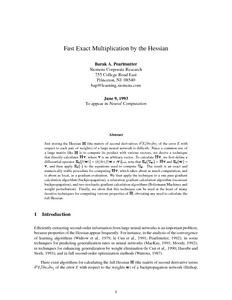# Fast Exact Multiplication by the Hessian

Pearlmutter, Barak A. (1994) Fast Exact Multiplication by the Hessian. Neural Computation, 6 (1). pp. 147-160. ISSN 0899-7667Previewmore...Add this article to your Mendeley library

## Abstract

Just storing the Hessian H (the matrix of second derivatives a2E/aw, aw, of the error E with respect to each pair of weights) of a large neural network is difficult. Since a common use of a large matrix like H is to compute its product with various vectors, we derive a technique that directly calculates Hv, where v is an arbitrary vector. To calculate Hv, we first define a differential operator Rycf (w)}=(a/&) f (w+ W) J~=~, note that%{Vw}= Hv and%{w}= v, and then apply R {.} to the equations used to compute 0,.

Item Type: Article Fast Exact Multiplication; Hessian; Faculty of Science and Engineering > Computer ScienceFaculty of Science and Engineering > Research Institutes > Hamilton Institute 5501 Barak Pearlmutter 15 Oct 2014 10:21 Neural Computation MIT Press YesItem control page